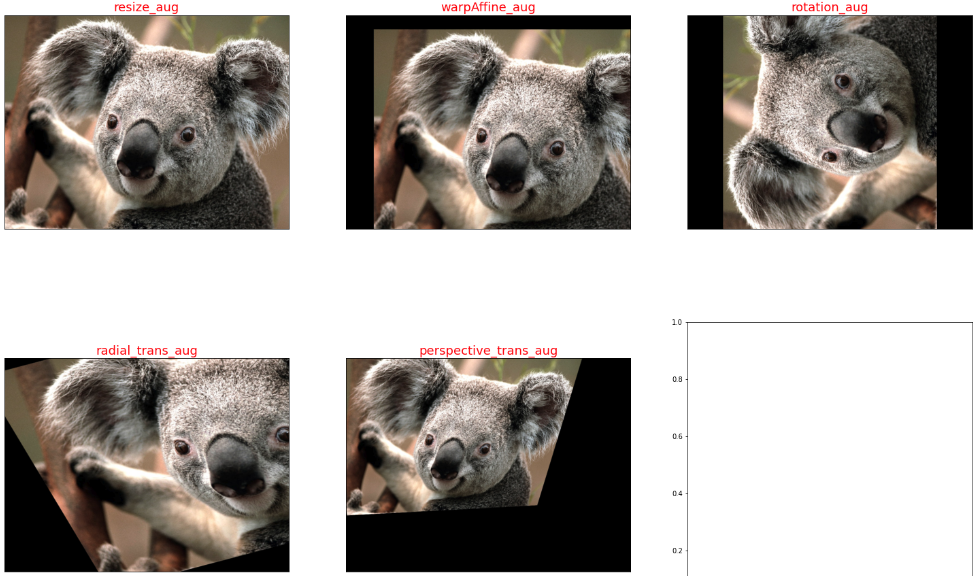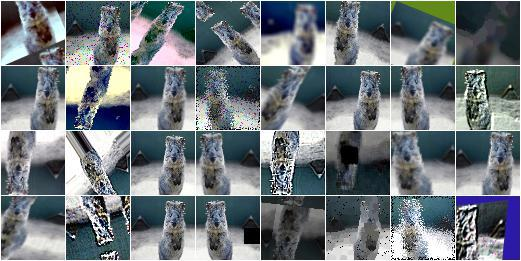# #百人创作先锋团#深度学习炼丹-数据增强 原创

0收藏

## 一，数据增强概述

HSV 表达彩色图像的方式由三个部分组成：
Hue（色调、色相）
Saturation（饱和度、色彩纯净度）
Value（明度）

• 线下增强（offline augmentation）: 适用于较小的数据集（smaller dataset）。
• 线上增强（online augmentation）: 适用于较大的数据集（larger datasets）。

## 二，opencv 图像增强-几何变换

OpenCV 提供的几何变换函数如下所示:

1，拓展缩放: 拓展缩放，改变图像的尺寸大小

cv2.resize(): 。常用的参数有设定图像尺寸、缩放因子和插值方法。

2，平移: 将对象换一个位置。

cv2.warpAffine(): 函数第一个参数是原图像，第二个参数是移动矩阵，第三个参数是输出图像大小 (width,height)。举例，如果要沿 $(x，y)$ 方向移动，移动的距离是 $(tx ，ty)$，则以下面的方式构建移动矩阵:
$$\begin{bmatrix} 1 & 0 & t_x\ 0 & 1 & t_y \end{bmatrix}$$

3，旋转: 对一个图像旋转角度 $\theta$。

getRotationMatrix2D 函数第一个参数为旋转中心，第二个为旋转角度，第三个为旋转后的缩放因子

4，放射变换（也叫平面变换/径向变换）: 在仿射变换中，原图中所有的平行线在结果图像中依旧平行。

5，透视变换（也叫空间变换）: 转换之后，直线仍是直线。

import cv2
import matplotlib.pyplot as plt
from PIL import Image
import numpy as np

def show_images(imgs, num_rows, num_cols, titles=None, scale=8.5):
"""Plot a list of images.

Defined in :numref:sec_utils"""
figsize = (num_cols * scale, num_rows * scale)
_, axes = plt.subplots(num_rows, num_cols, figsize=figsize)
axes = axes.flatten()
for i, (ax, img) in enumerate(zip(axes, imgs)):
try:
img = np.array(img)
except:
pass
ax.imshow(img)
ax.axes.get_xaxis().set_visible(False)
ax.axes.get_yaxis().set_visible(False)
if titles:
ax.set_title(titles[i])
return axes

# Some Geometric Transformation of Images
class GeometricTransAug(object):
def __init__(self, image_path):
img = Image.open(image_path) # load the image
self.img_np = np.array(img) # convert PIL image to numpy array
self.rows, self.cols, self.ch = self.img_np.shape
self.geometry_trans_aug_visual(self.img_np)

def resize_aug(self, img_np):
# 直接设置了缩放因子, 缩放原大小的2倍
res = cv2.resize(img_np, None, fx=2, fy=2, interpolation = cv2.INTER_CUBIC)
return res

def warpAffine_aug(self, img_np):
# 先构建转换矩阵, 将图像像素点整体进行(100,50)位移：
M = np.float32([[1,0,100],[0,1,50]])
res = cv2.warpAffine(img_np, M,(self.cols, self.rows))
return res

def rotation_aug(self, img_np):
rows, cols, ch = img_np.shape
# 先构建转换矩阵,图像相对于中心旋转90度而不进行任何缩放。
M = cv2.getRotationMatrix2D((self.cols/2, self.rows/2), 90, 1)
res = cv2.warpAffine(img_np, M, (self.cols, self.rows))
return res

# 仿射变换需要从原图像中找到三个点以及他们在输出图像中的位置
pts1 = np.float32([[50,50],[200,50],[50,200]])
pts2 = np.float32([[10,100],[200,50],[100,250]])
# 通过 getAffineTransform 创建一个 2x3 的转换矩阵
M = cv2.getAffineTransform(pts1,pts2)

res = cv2.warpAffine(img_np, M, dsize = (self.cols, self.rows))
return res

def perspective_trans_aug(self, img_np):
# 透视变换需要一个 3x3 变换矩阵
pts1 = np.float32([[56,65],[368,52],[28,387],[389,390]])
pts2 = np.float32([[0,0],[300,0],[0,300],[300,300]])

M = cv2.getPerspectiveTransform(pts1,pts2)
# dsize: size of the output image.
res = cv2.warpPerspective(img_np, M, dsize = (300,300))

return res

def geometry_trans_aug_visual(self, img_np):
res1 = self.resize_aug(img_np)
res2 = self.warpAffine_aug(img_np)
res3 = self.rotation_aug(img_np)
res5 = self.perspective_trans_aug(img_np)
imgs = [res1, res2, res3, res4, res5]
aug_titles = ["resize_aug", "warpAffine_aug", "rotation_aug", "radial_trans_aug", "perspective_trans_aug"]
# show_images 函数前文已经给出，这里不再复制过来
axes = show_images(imgs, 2, 3, titles=aug_titles, scale=8.5)

if __name__ == '__main__':
img_path = 'Koalainputimage.jpeg'
geometry_trans_aug = GeometricTransAug(img_path)
img_np2 = geometry_trans_aug.img_np
print(img_np2.shape)## 三，pytorch 图像增强

pytorch 框架中，transforms 类提供了 22 个数据增强方法，对应代码在 transforms.py 文件中，它们既可以对 PIL Image 也能对 torch.*Tensor 数据类型进行增强。

api 的详细介绍可以参考官网文档-Transforming and augmenting images。本章只对 transforms 的 22 个方法进行简要介绍和总结。

1，裁剪-Crop

• 中心裁剪: transforms.CenterCrop
• 随机裁剪: transforms.RandomCrop
• 随机长宽比裁剪: transforms.RandomResizedCrop
• 上下左右中心裁剪: transforms.FiveCrop
• 上下左右中心裁剪后翻转: transforms.TenCrop

2，翻转和变换-Flip and Rotations

• 依概率 p 水平翻转:transforms.RandomHorizontalFlip(p=0.5)
• 依概率 p 垂直翻转:transforms.RandomVerticalFlip(p=0.5)
• 随机旋转:transforms.RandomRotation

3，图像变换

• resize: transforms.Resize
• min-max Normalization: 对应 torchvision.transforms.ToTensor() 方法
• zero-mean Normalization: 对应 torchvision.transforms.Normalize() 方法
• 修改亮度、对比度和饱和度:transforms.ColorJitter
• 转灰度图: transforms.Grayscale
• 线性变换: transforms.LinearTransformation()
• 仿射变换: transforms.RandomAffine
• 依概率 p 转为灰度图: transforms.RandomGrayscale
• 将数据转换为 PILImage: transforms.ToPILImage
• transforms.Lambda: Apply a user-defined lambda as a transform.

4，对 transforms 操作，使数据增强更灵活

• transforms.RandomChoice(transforms): 从给定的一系列 transforms 中选一个进行操作
• transforms.RandomApply(transforms, p=0.5): 给一个 transform 加上概率，依概率进行操作
• transforms.RandomOrder: 将 transforms 中的操作随机打乱

# 为了节省空间，这里不再列出导入相应库的代码和show_images函数
img_PIL = Image.open('astronaut.jpeg')
print(img_PIL.size)
# if you change the seed, make sure that the randomly-applied transforms
# properly show that the image can be both transformed and *not* transformed!
torch.manual_seed(0)
# size 参数: desired output size.
resized_imgs = [transforms.Resize(size=size)(orig_img) for size in (30, 50, 100, orig_img.size)]
show_images(resized_imgs, 1, 4)## 四，imgaug 图像增强

imgaug 是一个用于机器学习实验中图像增强的库。 它支持广泛的增强技术，允许轻松组合这些技术并以随机顺序或在多个 CPU 内核上执行它们，具有简单而强大的随机接口，不仅可以增强图像，还可以增强关键点/地标、边界框、 热图和分割图。imgaug 的图像增强方法如下所示。

• Basics
• Keypoints
• Bounding Boxes
• Heatmaps
• Stochastic Parameters: 随机参数
• Blending/Overlaying images: 混合/叠加图像
• Augmenters: 增强器概述

## 参考资料

1. 《解析卷积神经网络-第5、6章》
2. 《OpenCV-Python-Toturial-中文版》